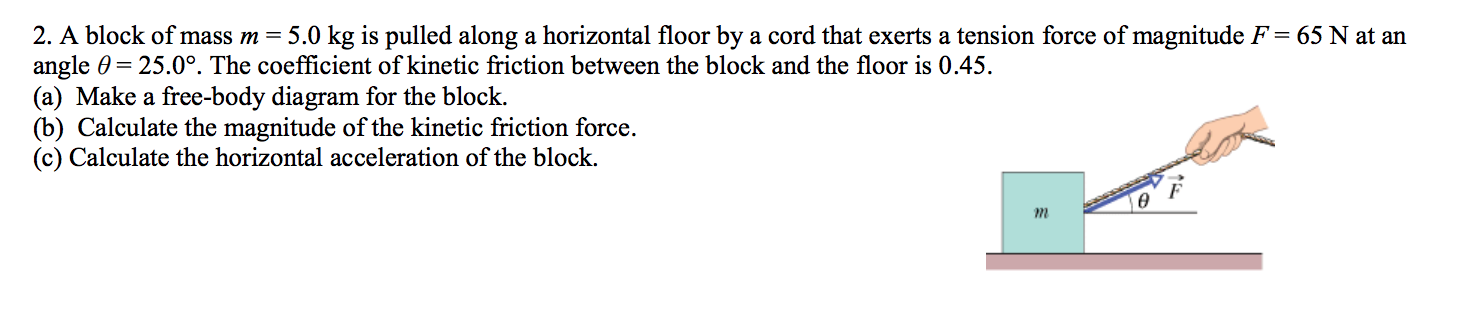# 2. A block of mass m = 5.0 kg is pulled along a horizontal floor by a cord that exerts a tension force of magnitude F = 65 N at anangle 0 25.0°. The coefficient of kinetic friction between the block and the floor is 0.45(a) Make a free-body diagram for the block(b) Calculate the magnitude of the kinetic friction force.(c) Calculate the horizontal acceleration of the block.F

Question
33 viewshelp_outlineImage Transcriptionclose2. A block of mass m = 5.0 kg is pulled along a horizontal floor by a cord that exerts a tension force of magnitude F = 65 N at an angle 0 25.0°. The coefficient of kinetic friction between the block and the floor is 0.45 (a) Make a free-body diagram for the block (b) Calculate the magnitude of the kinetic friction force. (c) Calculate the horizontal acceleration of the block. F fullscreen
check_circle

Step 1

The free body diagram is shown above.

Step 2

(b)Write the expression of force along the vertical.

Step 3

Write the expression for kine...

### Want to see the full answer?

See Solution

#### Want to see this answer and more?

Solutions are written by subject experts who are available 24/7. Questions are typically answered within 1 hour.*

See Solution
*Response times may vary by subject and question.
Tagged in

### Kinematics